Open in App
Not now

# Shopping Cart Project Using C Language

• Last Updated : 17 Jan, 2023

Online Shopping applications are one of the favorite applications out of all online applications. It is a very much used application in everyone’s life. So, Let’s try to create a Shopping cart using C language which can perform basic tasks for us.

### The functionality of the Shopping Cart

Although online Shopping has many functionalities we can’t include all of them. So, a few Functionalities of the shopping cart can use are mentioned below:

1. Signup
3. Search items
4. Bill

### Approach and Functionality

#### Signup Functionality

This is the main function for a signup, Here we will check whether the account exists or not if not we will create a new account Following are the functionalities: Inputs are username, age, email, password, confirm the password, and mobile number.
Validate the inputs input data should be valid otherwise account will not create. After successful signup, you will be directly redirected to the login page

This function implements the login features of our project. While login in, the Email, and Password are validated and checked whether it is already signed up. After the successful login, there is an option to choose either Search_by_items or search by shops.

#### Order by Shop

In this functionality, the item is placed after selecting any shop. Once the shop is selected the list of items is displayed with their respective costs. Once the item is selected, you need to go to the option Select Cart for the successful ordering of the items.

#### Cart

This function will show you the Total cost of your order

### Data Input Conditions

Conditions for the data to be input on Sign up page are mentioned below:

1. Name: must contain the alphabet.
2. Age: must be greater than and not equal to 0.
3. Email: must contain @, a comma, and length should be greater than 5.
4. Passwords: must contain lengths between 8 to 12 with at least one uppercase, lowercase, number, and special character. Both password and confirm password should be the same.
5. Mobile numbers: should contain numbers and exactly 10 digits.

Main Program:

## C

 `// C Program to implement``// Shopping Cart``#include ``#include ``#include ``#include ``#include `` ` ` ` `// Structure declared for storing``// details``struct` `details {``    ``char` `uname;``    ``int` `age;``    ``char` `password;``    ``char` `email;``    ``char` `mobile;``};`` ` `// Structure to store details about``// shops``struct` `Shops {``    ``char` `shop;``    ``char` `item1;``    ``char` `item2;``    ``char` `item3;``    ``char` `item4;``    ``int` `first, second, third, fourth;``};`` ` `// Array of Structure declared``struct` `Shops m;``struct` `details s;`` ` `void` `signup();`` ` `void` `account_validate();``int` `validate();``void` `login();``void` `cart();``void` `shop();``void` `items();``void` `item_order(``int` `item);`` ` `void` `shop_initialize();``void` `Shop(``int` `shop_choice);`` ` `// Global variables declared``char` `t_name, t_password1;``char` `t_password2, t_email;``char` `t_mobile;``int` `flag = 1, i, j = 0, count = 0, caps = 0;``int` `Small = 0,total = 0 ,success = 0,special = 0, numbers = 0;``int` `x, choice,t_age,  item_choice, n,shop_choice, search_choice, confirm,ch, item, shop_id;`` ` ` ` `// Driver code``int` `main()``{``    ``// Loop while till which runs till break is called``    ``while` `(1) {`` ` `        ``// First Page``        ``printf``(``"\n\n\t******************Welcome to Shop "``               ``"Cart*******************\n"``);``        ``printf``(``"\n\n1)SIGNUP"``);``        ``printf``(``"\n2)LOGIN"``);``        ``printf``(``"\n3)EXIT"``);`` ` `        ``// Asking for choice``        ``printf``(``"\n\n\nEnter your choice : "``);``        ``scanf``(``"%d"``, &choice);`` ` ` ` `        ``// Switch used to check the input``        ``// choice``        ``switch` `(choice) {``        ``case` `1: {``            ``// Signup function called``            ``signup();``            ``break``;``        ``}``        ``case` `2: {``            ``// Login function called``            ``login();``            ``break``;``        ``}``        ``case` `3: {``            ``printf``(``                ``"\n\t*************************Thank you "``                ``"Visit Again***********************\n\n"``);`` ` `            ``// close the program``            ``return` `0;``        ``}``        ``default``: {`` ` `            ``// Choice entered is not correct``            ``printf``(``"\n\nPlease enter valid choice!!\n"``);``            ``break``;``        ``}``        ``}``    ``}``}`` ` ` ` `// Signup function declared``void` `signup()``{`` ` `    ``printf``(``"\n\n\t******************"``           ``"Welcome to Signup "``           ``"Page****************"``           ``"*\n\n"``);`` ` `    ``// Taking name as input``    ``printf``(``"\tEnter Your name : "``);``    ``scanf``(``"%s"``, t_name);`` ` `    ``// Taking email as input``    ``printf``(``"\tEnter Your Email : "``);``    ``scanf``(``"%s"``, t_email);`` ` `    ``// Taking password as input``    ``printf``(``"\tEnter Password : "``);``    ``scanf``(``"%s"``, t_password1);`` ` `    ``// Taking Confirm Password as input``    ``printf``(``"\tConfirm Password : "``);``    ``scanf``(``"%s"``, t_password2);`` ` `    ``// Taking Mobile number as input``    ``printf``(``"\tEnter Your Mobile Number : "``);``    ``scanf``(``"%s"``, t_mobile);`` ` `    ``// Taking Age as input``    ``printf``(``"\tEnter Your Age : "``);``    ``scanf``(``"%d"``, &t_age);`` ` `    ``x = validate();``    ``if` `(x == 1) {`` ` `        ``// Calling account_validate to check``        ``// if entered details follows the defined``        ``// rules``        ``account_validate();`` ` `        ``// login function appears``        ``login();``    ``}``}`` ` `// Signup process``int` `validate()``{``    ``// Name Validation``    ``for` `(i = 0; t_name[i] != ``'\0'``; i++) {``        ``if` `(!((t_name[i] >= ``'a'` `&& t_name[i] <= ``'z'``)``              ``|| (t_name[i] >= ``'A'` `&& t_name[i] <= ``'Z'``))) {``            ``printf``(``"\nPlease enter the valid Name!\n"``);``            ``flag = 0;``            ``break``;``        ``}``    ``}``    ``if` `(flag == 1) {``        ``count = 0;``        ``// Email ID Validation``        ``for` `(i = 0; t_email[i] != ``'\0'``; i++) {``            ``if` `(t_email[i] == ``'@'` `|| t_email[i] == ``'.'``)``                ``count++;``        ``}``        ``if` `(count >= 2 && ``strlen``(t_email) >= 5) {``            ``// Password Validation``            ``if` `(!``strcmp``(t_password1, t_password2)) {``                ``if` `(``strlen``(t_password1) >= 8``                    ``&& ``strlen``(t_password1) <= 12) {``                    ``caps = 0;``                    ``Small = 0;``                    ``numbers = 0;``                    ``special = 0;``                    ``for` `(i = 0; t_password1[i] != ``'\0'``;``                         ``i++) {``                        ``if` `(t_password1[i] >= ``'A'``                            ``&& t_password1[i] <= ``'Z'``)``                            ``caps++;``                        ``else` `if` `(t_password1[i] >= ``'a'``                                 ``&& t_password1[i] <= ``'z'``)``                            ``Small++;``                        ``else` `if` `(t_password1[i] >= ``'0'``                                 ``&& t_password1[i] <= ``'9'``)``                            ``numbers++;``                        ``else` `if` `(t_password1[i] == ``'@'``                                 ``|| t_password1[i] == ``'&'``                                 ``|| t_password1[i] == ``'#'``                                 ``|| t_password1[i] == ``'*'``)``                            ``special++;``                    ``}``                    ``if` `(caps >= 1 && Small >= 1``                        ``&& numbers >= 1 && special >= 1) {``                        ``// Age Validation``                        ``if` `(t_age != 0 && t_age > 0) {``                            ``// Mobile Validation``                            ``if` `(``strlen``(t_mobile) == 10) {``                                ``for` `(i = 0; i < 10; i++) {``                                    ``if` `(t_mobile[i] >= ``'0'``                                        ``&& t_mobile[i]``                                               ``<= ``'9'``) {``                                        ``success = 1;``                                    ``}``                                    ``else` `{``                                        ``printf``(``                                            ``"\n\nPlease"``);``                                        ``printf``(``                                            ``"Enter Valid "``);``                                        ``printf``(``                                            ``"Mobile "``                                            ``"Number\n\n"``);``                                        ``return` `0;``                                        ``break``;``                                    ``}``                                ``}`` ` `                                ``// Success flag to know``                                ``// every inputs are valid``                                ``if` `(success == 1)``                                    ``return` `1;``                            ``}``                            ``else` `{``                                ``printf``(``"\n\nPlease Enter "``                                       ``"the 10 digit "``                                       ``"mobile number\n\n"``);``                                ``return` `0;``                            ``}``                        ``}``                        ``else` `{``                            ``printf``(``"\n\nPlease Enter the "``                                   ``"valid age\n\n"``);``                            ``return` `0;``                        ``}``                    ``}``                    ``else` `{``                        ``printf``(``                            ``"\n\nPlease Enter the strong "``                            ``"password, Your password must "``                            ``"contain atleast one "``                            ``"uppercase,Lowercase, Number "``                            ``"and special character\n\n "``);``                        ``return` `0;``                    ``}``                ``}``                ``else` `{``                    ``printf``(``"\nYour Password is very "``                           ``"short\nLength must  between 8 "``                           ``"to 12\n\n"``);``                    ``return` `0;``                ``}``            ``}``            ``else` `{``                ``printf``(``"\nPassword Mismatch\n\n"``);``                ``return` `0;``            ``}``        ``}``        ``else` `{``            ``printf``(``"\nPlease Enter Valid E-Mail\n\n"``);``            ``return` `0;``        ``}``    ``}``}`` ` `// Account Validation``void` `account_validate()``{``    ``for` `(i = 0; i < 100; i++) {``        ``// Check account already exist``        ``if` `(!(``strcmp``(t_email, s[i].email)``              ``&& ``strcmp``(t_password1, s[i].password))) {``            ``printf``(``"\n\nAccount Already Existed. Please "``                   ``"Login !\n\n"``);``            ``login();``            ``break``;``        ``}``    ``}`` ` `    ``// If no account present it creates``    ``if` `(i == 100) {``        ``strcpy``(s[j].uname, t_name);``        ``s[j].age = t_age;``        ``strcpy``(s[j].password, t_password1);``        ``strcpy``(s[j].email, t_email);``        ``strcpy``(s[j].mobile, t_mobile);``        ``j++;``        ``printf``(``"\n\n\nAccount Successfully Created!\n\n"``);``    ``}``}`` ` `// Login function``void` `login()``{``    ``printf``(``"\n\n\t******************"``           ``"Welcome to Login "``           ``"Page********************"``           ``"****\n\n"``);``    ``printf``(``"\n\nLOGIN\n\n"``);``    ``printf``(``"\t Enter Your Email: "``);`` ` `    ``// Askinf for email``    ``scanf``(``"%s"``, t_email);``    ``printf``(``"\t Enter Your Password: "``);`` ` `    ``// Asking for your Password``    ``scanf``(``"%s"``, t_password1);`` ` `    ``for` `(i = 0; i < 100; i++) {``        ``// Check whether the input email is already existed``        ``// or not``        ``if` `(!``strcmp``(s[i].email, t_email)) {``            ``// Check whether the password is matched with``            ``// the email or not``            ``if` `(!``strcmp``(s[i].password, t_password1)) {``                ``printf``(``"\n\nWelcome %s, "``, s[i].uname);``                ``printf``(``                    ``"Your are successfully logged in\n\n "``);``                ``printf``(``                    ``"We Provide two ways of search : \n "``);``                ``printf``(``"1) Search By Shop\n "``);``                ``printf``(``"2) Search by item\n "``);``                ``printf``(``"3) Exit\n\n"``);``                ``printf``(``"Please Enter your choice : "``);``                ``scanf``(``"%d"``, &search_choice);`` ` `                ``// Getting the input whether``                ``// the user are going to search``                ``// /Order by Shop or search/``                ``// order by item.``                ``switch` `(search_choice) {``                ``case` `1: {``                    ``shop();``                    ``break``;``                ``}``                ``case` `2: {``                    ``items();``                    ``break``;``                ``}``                ``case` `3: {``                    ``// main function called again``                    ``main();``                    ``break``;``                ``}``                ``default``: {``                    ``// Choice entered is wrong``                    ``printf``(``"Please Enter the valid "``                           ``"choice!!!\n\n"``);``                    ``break``;``                ``}``                ``}``                ``break``;``            ``}``            ``else` `{``                ``// Password entered is wrong``                ``printf``(``"\n\nInvalid Password!!\n "``);``                ``printf``(``"Please Enter the correct "``                       ``"password\n\n"``);``                ``login();``                ``break``;``            ``}``        ``}``        ``else` `{``            ``// If details are incorrect``            ``printf``(``"\n\nAccount doesn't exist, Please "``                   ``"signup!!\n\n "``);``            ``main();``            ``break``;``        ``}``    ``}``}`` ` ` ` `// Calling shop_initialize function``void` `shop_initialize()``{``    ``// All the Products avaiable are being stored``    ``// in variables``    ``strcpy``(m.shop, ``"Puma"``);``    ``strcpy``(m.item1, ``"puma sneaker V2"``);``    ``strcpy``(m.item2, ``"mens dryflex"``);``    ``strcpy``(m.item3, ``"one8x puma"``);``    ``m.first = 3499;``    ``m.second = 2999;``    ``m.third = 5999;`` ` `    ``strcpy``(m.shop, ``"USA"``);``    ``strcpy``(m.item1, ``"US polo Tshirt"``);``    ``strcpy``(m.item2, ``"Shoes"``);``    ``strcpy``(m.item3, ``"shirt"``);``    ``m.first = 999;``    ``m.second = 2000;``    ``m.third = 1499;`` ` `    ``strcpy``(m.shop, ``"WROGN"``);``    ``strcpy``(m.item1, ``"Mens Watch"``);``    ``strcpy``(m.item2, ``"mens solid jacket"``);``    ``strcpy``(m.item3, ``"casusal T-shirt"``);``    ``m.first = 2000;``    ``m.second = 2239;``    ``m.third = 799;``}`` ` `// shop Function is called``void` `shop()``{``    ``// Shop Products are collected``    ``shop_initialize();`` ` `    ``// Showing all the availableshop``    ``printf``(``"\n\nPlease Choose the Shop \n\n1) %s\n2) "``           ``"%s\n3) %s"``,``           ``m.shop, m.shop, m.shop);``    ``printf``(``"\n4) Exit\n\nPlease "``);``    ``printf``(``"select the shop\t"``);`` ` `    ``// Asking for choice``    ``scanf``(``"%d"``, &shop_choice);``    ``if` `(shop_choice > 4) {``        ``printf``(``"Please Enter the"``);``        ``printf``(``"valid choice\n\n"``);``        ``shop();``    ``}``    ``else` `if` `(shop_choice == 4)``        ``main();``    ``else``{``        ``// Shop function called with your choice``        ``Shop(shop_choice);``    ``}``}`` ` `// Function to implement the shop``void` `Shop(``int` `shop_choice)``{``    ``// total variable``    ``total = 0;`` ` `    ``while` `(1) {`` ` `        ``printf``(``"\n\nList of items available "``);`` ` `        ``printf``(``"in %s\n\n1) %s --> %d\n"``,``               ``m[shop_choice].shop, m[shop_choice].item1,``               ``m[shop_choice].first);`` ` `        ``printf``(``"2) %s --> %d\n3) %s --> %d\n"``,``               ``m[shop_choice].item2, m[shop_choice].second,``               ``m[shop_choice].item3, m[shop_choice].third);`` ` `        ``printf``(``"4) Cart\n5) Exit\n\nPlease Enter "``);``        ``printf``(``"Your Choice : "``);``        ``scanf``(``"%d"``, &item_choice);`` ` `        ``// Get the input for no of items to calculate the``        ``// total amount``        ``if` `(item_choice == 1) {``            ``printf``(``"Please Enter the "``);``            ``printf``(``"Count of %s\t"``, m[shop_choice].item1);``            ``scanf``(``"%d"``, &n);``            ``total = total + (n * m[shop_choice].first);``        ``}``        ``else` `if` `(item_choice == 2) {``            ``printf``(``"Please Enter the Count"``);``            ``printf``(``"of %s : "``, m[shop_choice].item1);``            ``scanf``(``"%d"``, &n);``            ``total = total + (n * m[shop_choice].second);``        ``}``        ``else` `if` `(item_choice == 3) {``            ``printf``(``"Please Enter the Count"``);``            ``printf``(``"of %s : "``, m[shop_choice].item3);``            ``scanf``(``"%d"``, &n);``            ``total = total + (n * m[shop_choice].third);``        ``}`` ` `        ``else` `if` `(item_choice == 4) {``            ``cart();``        ``}``        ``else` `if` `(item_choice == 5) {``            ``shop();``        ``}``        ``else` `{``            ``printf``(``"Please Enter the"``);``            ``printf``(``"valid Choice\n\n"``);``        ``}``    ``}``}`` ` `void` `items()``{``    ``total = 0;``    ``// Initialize all the Shop and their items``    ``shop_initialize();``    ``while` `(1) {`` ` `        ``//Showing all the choices of items``        ``printf``(``"\n\nPlease choose the "``);``        ``printf``(``"item\n\n1) %s /t--> %d\n2) %s/t --> %d"``,``               ``m.item1, m.first, m.item2,``               ``m.second);`` ` `        ``printf``(``"\n3) %s/t --> %d\n4) %s/t --> %d\n"``,``               ``m.item3, m.third, m.item1,``               ``m.first);`` ` `        ``printf``(``"5) %s/t --> %d\n6) %s/t --> %d\n"``,``               ``m.item2, m.second, m.item3,``               ``m.third);`` ` `        ``printf``(``"7) %s/t --> %d\n8) %s/t --> %d\n"``,``               ``m.item1, m.first, m.item2,``               ``m.second);`` ` `        ``printf``(``"9) %s/t --> %d\n10) Cart\n"``, m.item3,``               ``m.third);``        ``printf``(``"11) Exit"``);``        ``printf``(``"\nPlease Enter Your Choice : "``);``        ``scanf``(``"%d"``, &item);``        ``if` `(item > 10) {``            ``printf``(``"Please Enter the "``);``            ``printf``(``"valid choice\n\n"``);``            ``items();``        ``}``        ``// Moves to the cart``        ``else` `if` `(item == 10)``            ``cart();``        ``else` `if` `(item == 11)``            ``// exit(1);``            ``return``;``        ``// Function to get the no of items and to calculate``        ``// the total amount of the order.``        ``else``            ``item_order(item);``    ``}``}`` ` `// item_order function to process items ordered``void` `item_order(``int` `item)``{`` ` `    ``if` `(item >= 1 && item <= 3)``        ``shop_id = 1;``    ``else` `if` `(item >= 4 && item <= 6)``        ``shop_id = 2;``    ``else``        ``shop_id = 3;``    ``if` `((item % 3) == 1) {``        ``// Asking for choice of product``        ``printf``(``"Please Enter the"``);``        ``printf``(``" Count of %s : "``, m[shop_id].item1);``        ``scanf``(``"%d"``, &n);``        ``total = total + (n * m[shop_id].first);``    ``}``    ``else` `if` `((item % 3) == 2) {``        ``// Asking for choice of product``        ``printf``(``"Please Enter the "``);``        ``printf``(``"Count of %s\t"``, m[shop_id].item2);``        ``scanf``(``"%d"``, &n);``        ``total = total + (n * m[shop_id].second);``    ``}``    ``else` `if` `((item % 3) == 0) {``        ``// Asking for choice of product``        ``printf``(``"Please Enter the Count of %s\t"``,``               ``m[shop_id].item3);``        ``scanf``(``"%d"``, &n);``        ``total = total + (n * m[shop_id].third);``    ``}``}`` ` `// Function to implement the cart``void` `cart()``{``    ``// details about the products in cart``    ``printf``(``"\n\n\n\n\t*********************************"``           ``"Cart*********************************"``);``    ``printf``(``"\n\nYour Total Order Amount is : %d\n"``, total);``    ``printf``(``"\n\nDo You wish to order (y=1/n=0) : "``);``    ``scanf``(``"%d"``, &ch);``    ``if` `(ch == 1) {``        ``printf``(``"\n\nThank You for your Order"``);``        ``printf``(``"\nYour item is on the way. Welcome again "``               ``"\n\n "``);`` ` `        ``main();``        ``return``;``    ``}``    ``else` `if` `(ch == 0) {``        ``printf``(``"To cancel Your Order = 1 \nTo Exit = 0  "``);``        ``printf``(``"Select option : "``);``        ``scanf``(``"%d"``, &confirm);``        ``if` `(confirm == 1) {``            ``//Failed``            ``printf``(``"\n\nOops! Your item is cancelled!! "``                   ``"Exiting..\n\n"``);``            ``printf``(``"Thank You visit again!\n"``);``            ``main();``            ``return``;``        ``}``        ``else` `{``            ``// Product is bought``            ``printf``(``"\n\n\t\t************Thank "``                   ``"You******************\n\n"``);``            ``login();``        ``}``    ``}``    ``else` `{``        ``// Asking for choice``        ``printf``(``"\n\nPlease Enter the correct choice\n\n "``);``        ``cart();``    ``}``}`

Output:

#### Welcome Page: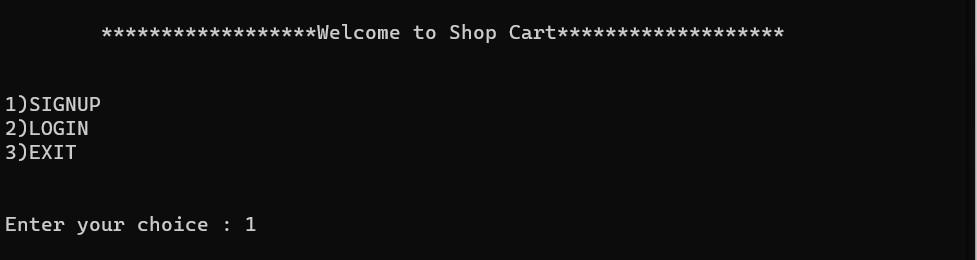First Page of C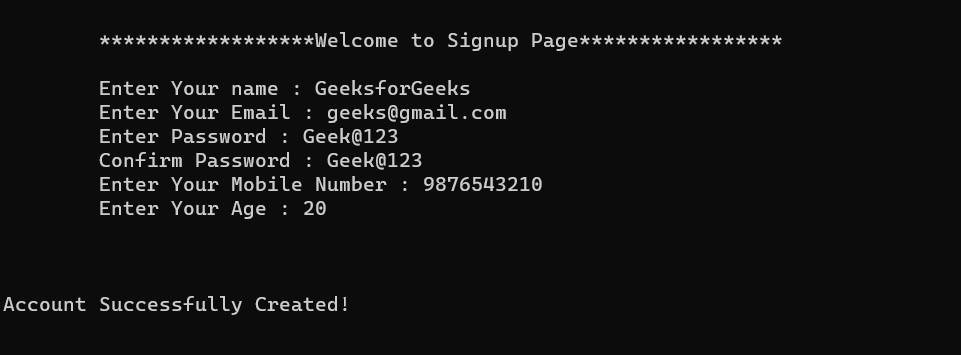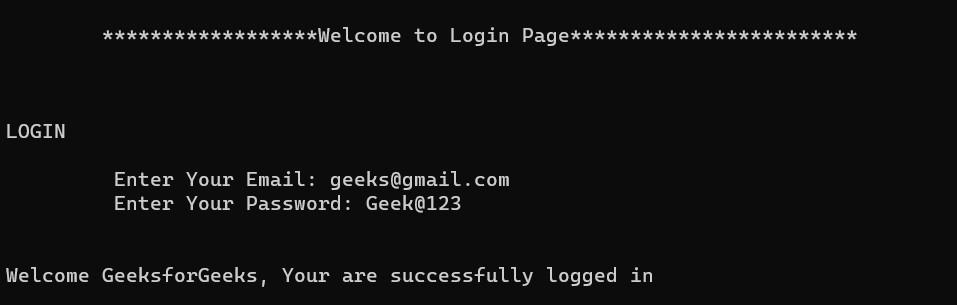#### List of Shops and Items: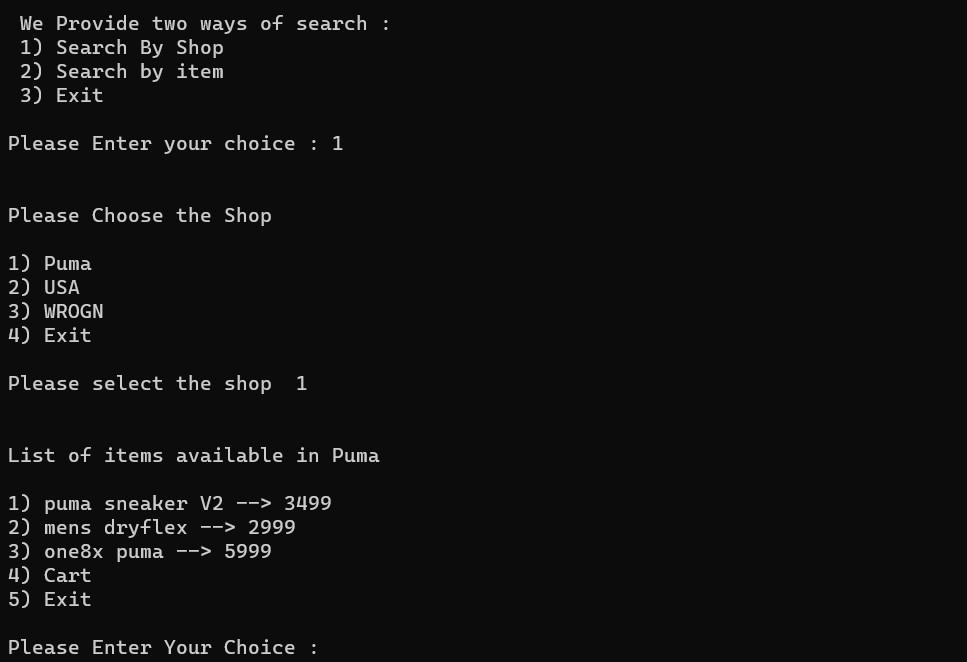List of Shops and Items

#### Bill Generated: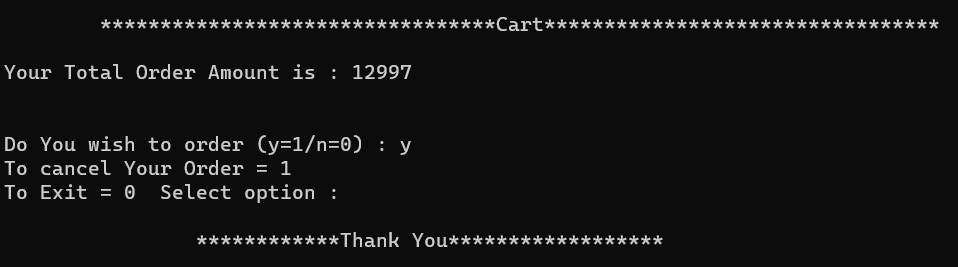Bill Generated

My Personal Notes arrow_drop_up